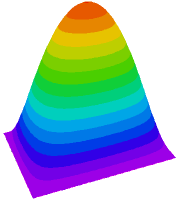﻿ Sample Problems > Applications > Stress > free_plate

# free_plate

Navigation:  Sample Problems > Applications > Stress >

# free_plate{ FREE_PLATE.PDE

This example considers the bending of a thin rectangular plate under a

For small displacements, the deflection U is described by the Biharmonic

equation of plate flexure

del2(del2(U)) + Q/D  =  0

where

D = E*h^3/(12*(1-nu^2))

E is Young's Modulus

nu is Poisson's ratio

and   h is the plate thickness.

The boundary conditions to be imposed depend on the way in which the

plate is mounted.  Here we consider the case of a simply supported

boundary, for which the correct conditions are

U = 0

Mn = 0

where Mn is the tangential component of the bending moment, which in turn

is related to the curvature of the plate. An approximation to the second

boundary condition is then

del2(U) = 0.

FlexPDE cannot directly solve the fourth order equation, but if we

define V = del2(U), then the deflection equation becomes

del2(U) = V

del2(V) + Q = 0

with the boundary conditions

U = 0

V = 0.

The particular problem addressed here is a plate of 16-gauge steel,

8 x 11.2 inches, covering a vacuum chamber, with atmospheric pressure

problem are readily available, for example in Roark's Formulas for Stress

& Strain, from which the maximum deflection is Umax =  0.746, as compared

with the FlexPDE result of 0.750.

(See FIXED_PLATE.PDE for the solution with a clamped edge.)

Note: Care must be exercised when extending this formulation to more complex

problems.  In particular, in the equation del2(U) = V, V acts as a source

in the boundary-value equation for U.  Imposing a value boundary condition

on U does not enforce V = del2(U).

}

Title " Plate Bending - simple support "

Select

ngrid=10                   { increase initial gridding }

cubic           { Use Cubic Basis }

Variables

U(0.1)

V(0.1)

Definitions

xslab = 11.2

yslab = 8

h = 0.0598 {16 ga}

L = 1.0e6

E = 29e6

Q = 14.7

nu = .3

D = E*h^3/(12*(1-nu^2))

 Initial Values    U =  0    V =  0 Equations     U: del2(U) = V     V: del2(V) = Q/D Boundaries   Region 1     start (0,0)     value(U) = 0     value(V) = 0     line to (xslab,0)           to (xslab,yslab)           to (0,yslab)           to close Monitors   contour(U)Plots

contour (U) as "Displacement"

elevation(U) from (0,yslab/2) to (xslab,yslab/2) as "Displacement"

surface(U) as "Displacement"

End